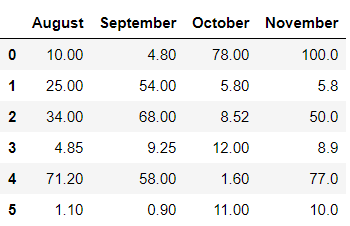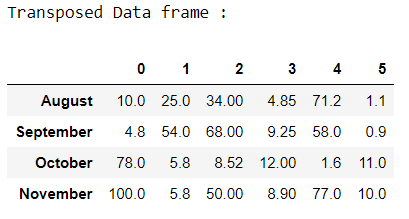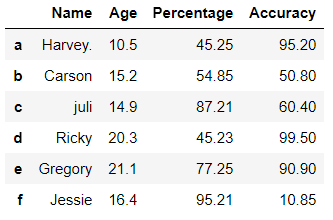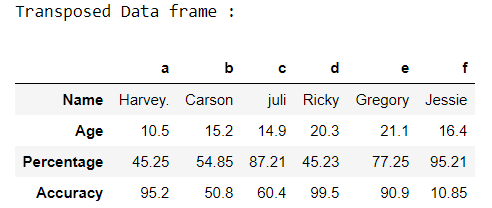Open in App
Not now

# pandas.DataFrame.T() function in Python

• Last Updated : 11 Oct, 2020

pandas.DataFrame.T property is used to transpose index and columns of the data frame. The property T is somehow related to method transpose().  The main function of this property is to create a reflection of the data frame overs the main diagonal by making rows as columns and vice versa.

Syntax: DataFrame.T

Parameters:
copy: If True, the underlying data is copied, otherwise (default).

Returns: The Transposed data frame

Example 1:

Sometimes we need to transpose the data frame in order to study it more accurately. In this situation pandas.DataFrame.T property plays an important role.

## Python3

 `# Importing pandas module ` `import` `pandas as pd ` ` `  `# Creating a dictionary ` `dit ``=` `{``'August'``: [``10``, ``25``, ``34``, ``4.85``, ``71.2``, ``1.1``], ` `       ``'September'``: [``4.8``, ``54``, ``68``, ``9.25``, ``58``, ``0.9``], ` `       ``'October'``: [``78``, ``5.8``, ``8.52``, ``12``, ``1.6``, ``11``], ` `       ``'November'``: [``100``, ``5.8``, ``50``, ``8.9``, ``77``, ``10``]} ` ` `  `# Converting it to data frame ` `df ``=` `pd.DataFrame(data``=``dit) ` ` `  `# Original DataFrame ` `df `

Output:Transposing the data frame.

## Python3

 `# Transposing the data frame  ` `# using dataframe.T property ` `df_trans ``=` `df.T ` ` `  `print``(``"Transposed Data frame :"``) ` `df_trans`

Output:In the above example, we transpose the data frame ‘df’ having numeric values/content.

Example 2:

## Python3

 `# Import pandas library ` `import` `pandas as pd ` ` `  `# initialize list of lists ` `data ``=` `[[``'Harvey.'``, ``10.5``, ``45.25``, ``95.2``], ` `        ``[``'Carson'``, ``15.2``, ``54.85``, ``50.8``], ` `        ``[``'juli'``, ``14.9``, ``87.21``, ``60.4``], ` `        ``[``'Ricky'``, ``20.3``, ``45.23``, ``99.5``], ` `        ``[``'Gregory'``, ``21.1``, ``77.25``, ``90.9``], ` `        ``[``'Jessie'``, ``16.4``, ``95.21``, ``10.85``]] ` ` `  `# Create the pandas DataFrame ` `df ``=` `pd.DataFrame(data, columns``=``[``'Name'``, ``'Age'``, ``'Percentage'``, ``'Accuracy'``], ` `                  ``index``=``[``'a'``, ``'b'``, ``'c'``, ``'d'``, ``'e'``, ``'f'``]) ` ` `  `# print dataframe. ` `df`

Output:Transposing the dataframe.

## Python3

 `# Transposing the data frame  ` `# using dataframe.T property ` `df_trans ``=` `df.T ` ` `  `print``(``"Transposed Data frame :"``) ` `df_trans`

Output:In the above example, we transpose the data frame ‘df’ having mixed up data type.

My Personal Notes arrow_drop_up
Related Articles